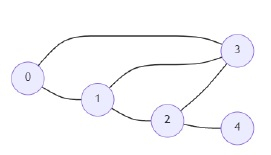# Program to find maximal network rank in Python

Suppose there are n cities and there are some roads connecting these cities. Each roads[i] = [u, v] indicates that there is a two-way road between cities u and v. Now consider network rank is the total number of directly connected roads to either city. When a road is directly connected to both cities, it is only counted once. And the maximal network rank of the network is the maximum network rank of all pairs of different cities. So, if we have different roads, we have to find the maximal network rank of the entire network.

So, if the input is likethen the output will be 5 because there are five different ways to connect cities 1 and 2

To solve this, we will follow these steps −

• n := number of nodes
• s := a new set
• d := a map, if some key is not present then return 0 for it
• for each edge (x,y) in roads, do
• d[x] := d[x] + 1
• d[y] := d[y] + 1
• insert pair (x,y) into d
• ans := 0
• l := a new list from range 0 to n
• sort l based on node numbers in descending order
• threshold := minimum of d[l] and d[l]
• for i in range 0 to size of l - 1, do
• for j in range i+1 to size of l - 1, do
• if d[l[j]] < threshold, then
• come out from loop
• curr := d[l[i]] + d[l[j]]
• if (l[i],l[j]) is present in s or (l[j],l[i]) is present in s, then
• curr := curr - 1
• ans := maximum of ans and curr
• return ans

## Example

Let us see the following implementation to get better understanding −

from collections import defaultdict
nodes = set()
s = set()
d = defaultdict(int)
nodes.update([x,y])
d[x]+=1
d[y]+=1

ans = 0
n = len(nodes)
l = list(range(n))
l.sort(key=lambda x:d[x], reverse = True)
threshold = min(d[l],d[l])
for i in range(len(l)-1):
for j in range(i+1,len(l)):
if d[l[j]]<threshold:
break
curr = d[l[i]]+d[l[j]]
if (l[i],l[j]) in s or (l[j],l[i]) in s:
curr-=1
ans = max(ans,curr)
return ans

print(solve(roads))
[(0,1),(0,3),(1,2),(1,3),(2,3),(2,4)]
5